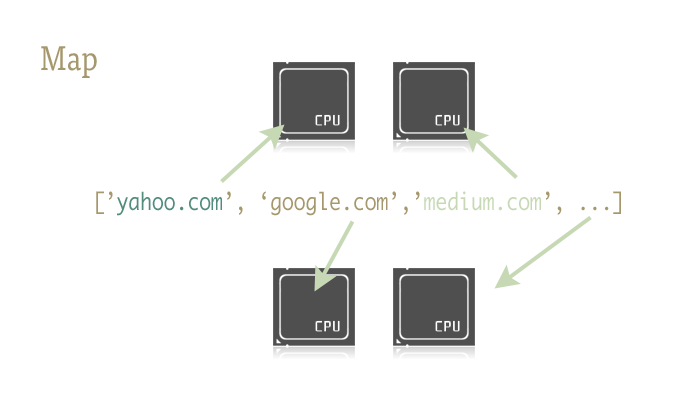## 一行式并行方案（译）

#### 沿袭的例子

``````# coding: utf-8
# Example.py
'''

'''

import time
import Queue

def __init__(self, queue):
self._queue = queue

def run(self):
while True:
# queue.get() 会阻塞当前线程，直到获取到一个数据项
msg = self._queue.get()
# 检查当前消息是否是个“毒药丸”
if isinstance(msg, str) and msg == 'quit':
# 如果是，则退出循环
break
# “处理” (这里是打印)从队列中取出的数据项
# 我始终是这么的友好
print 'Bye byes!'

def Producer():
# Queue用于在线程之间共享数据项
queue = Queue.Queue()

# 创建一个工作实例
worker = Consumer(queue)
# start方法会调用内部的run()方法来开启线程
worker.start()

# 变量，用于追踪开始的时间
start_time = time.time()
# 在5秒之内
while time.time() - start_time < 5:
# “生产”一块工作，放入队列中，由消费者来处理
queue.put('something at %s' % time.time())
# 睡眠一会儿，以避免过多的消息
time.sleep(1)

# 这是杀死线程的“毒药丸”方式
queue.put('quit')
# 等待线程关闭
worker.join()

if __name__ == '__main__':
Producer()
``````

#### 问题（我认为的）

``````# coding: utf-8
# Example2.py
'''

'''

import time
import Queue
import urllib2

def __init__(self, queue):
self._queue = queue

def run(self):
while True:
content = self._queue.get()
if isinstance(content, str) and content == 'quit':
break
response = urllib2.urlopen(content)
print 'Bye byes!'

def Producer():
urls = [
'http://www.python.org', 'http://www.yahoo.com'
# 等等...
]
queue = Queue.Queue()
start_time = time.time()

# 加入待处理的URL
for url in urls:
queue.put(url)
# 加入毒药丸
queue.put('quit')
worker.join()

print 'Done! Time taken: {}'.format(time.time() - start_time)

def build_worker_pool(queue, size):
workers = []
for _ in range(size):
worker = Consumer(queue)
worker.start()
workers.append(worker)
return workers

if __name__ == '__main__':
Producer()
``````

## 引入：Map

Map是一个酷酷的小东西，也是在Python代码轻松引入并行的关键。对此不熟悉的人会认为map是从函数式语言（如Lisp）借鉴来的东西。map是一个函数 - 将另一个函数映射到一个序列上。例如：

``````urls = ['http://www.yahoo.com', 'http://www.reddit.com']
results = map(urllib2.urlopen, urls)
``````

``````results = []
for url in urls:
results.append(urllib2.urlopen(url))
``````

Map会为我们处理在序列上的迭代，应用函数，最后将结果存入一个方便使用的列表。Python标准库中multiprocessing模块，以及极少人知但同样出色的子模块multiprocessing.dummy，提供了map函数的并行版本。

Dummy是multiprocessing模块的精确克隆，唯一的区别是：multiprocessing基于进程工作，而dummy模块使用线程（也就带来了常见的Python限制）。因此，任何东西可套用到一个模块，也就可以套用到另一个模块。在两个模块之间来回切换也就相当容易，当你不太确定一些框架调用是IO密集型还是CPU密集型时，想做探索性质的编程，这一点会让你觉得非常赞！

#### 开始

``````# 以下两行引入其一即可
from multiprocessing import Pool
from multiprocessing.dummy import Pool as ThreadPool
``````

``````# 译注：这里其实是以dummy模块为例
``````

pool对象可以有若干参数，但目前，只需关注第一个：进程/线程数量。这个参数用于设置池中的工作者数目。如果留空，默认为机器的CPU核数。

``````pool = ThreadPool(4) # 将池的大小设置为4
``````

``````import urllib2
from multiprocessing.dummy import Pool as ThreadPool

urls = [
'http://www.python.org',
'http://www.onlamp.com/pub/a/python/2003/04/17/metaclasses.html',
'http://www.python.org/doc/',
'http://www.python.org/getit/',
'http://www.python.org/community/',
'https://wiki.python.org/moin/',
'http://planet.python.org/',
'https://wiki.python.org/moin/LocalUserGroups',
'http://www.python.org/psf/',
'http://docs.python.org/devguide/',
'http://www.python.org/community/awards/'
# 等等...
]

# 创建一个工作者线程池
# 在各个线程中打开url，并返回结果
results = pool.map(urllib2.urlopen, urls)
#close the pool and wait for the work to finish
# 关闭线程池，等待工作结束
pool.close()
pool.join()
``````

``````# 译注：我觉得与串行处理方式对比意义不大，应该和队列的方式进行性能对比
results = []
for url in urls:
result = urllib2.urlopen(url)
results.append(result)

# # ------- 对比 ------- #

# # ------- 池的大小为4 ------- #
results = pool.map(urllib2.urlopen, urls)

# # ------- 池的大小为8 ------- #

results = pool.map(urllib2.urlopen, urls)

# # ------- 池的大小为13 ------- #

results = pool.map(urllib2.urlopen, urls)
``````

``````单线程: 14.4 秒

``````

## 现实中的Example 2

``````from multiprocessing import Pool
from PIL import Image

SIZE = (75,75)
SAVE_DIRECTORY = 'thumbs'

def get_image_paths(folder):
return (os.path.join(folder, f)
for f in os.listdir(folder)
if 'jpeg' in f)

def create_thumbnail(filename):
im = Image.open(filename)
im.thumbnail(SIZE, Image.ANTIALIAS)
base, fname = os.path.split(filename)
save_path = os.path.join(base, SAVE_DIRECTORY, fname)
im.save(save_path)

if __name__ == '__main__':
folder = os.path.abspath(
'11_18_2013_R000_IQM_Big_Sur_Mon__e10d1958e7b766c3e840')
os.mkdir(os.path.join(folder, SAVE_DIRECTORY))

images = get_image_paths(folder)

for image in images:
create_thumbnail(image)
``````

``````from multiprocessing import Pool
from PIL import Image

SIZE = (75,75)
SAVE_DIRECTORY = 'thumbs'

def get_image_paths(folder):
return (os.path.join(folder, f)
for f in os.listdir(folder)
if 'jpeg' in f)

def create_thumbnail(filename):
im = Image.open(filename)
im.thumbnail(SIZE, Image.ANTIALIAS)
base, fname = os.path.split(filename)
save_path = os.path.join(base, SAVE_DIRECTORY, fname)
im.save(save_path)

if __name__ == '__main__':
folder = os.path.abspath(
'11_18_2013_R000_IQM_Big_Sur_Mon__e10d1958e7b766c3e840')
os.mkdir(os.path.join(folder, SAVE_DIRECTORY))

images = get_image_paths(folder)

pool = Pool()
pool.map(create_thumbnail, images)
pool.close()
pool.join()
``````

5.6秒！